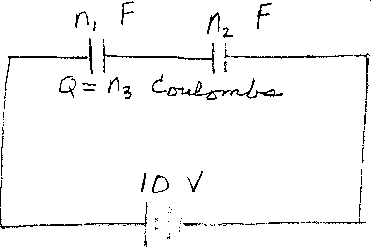Problem B4: Two capacitors are connected in series across a battery as shown in the figure above. One capacitor has a capacitance of n1 Farads, and the other has a capacitance of n2 Farads. If the voltage of the battery is 10 Volts, then how much charge collects on capacitor 1's plates? If the charge equals n3 Coulombs, then what is n3?n1 = n2 = Input n3:
If you are currently in my class, you can record your grade by entering your name and student ID number (without the leading zeros) below and clicking on "record grade".
 First Name = Last Name = ID = Problem: